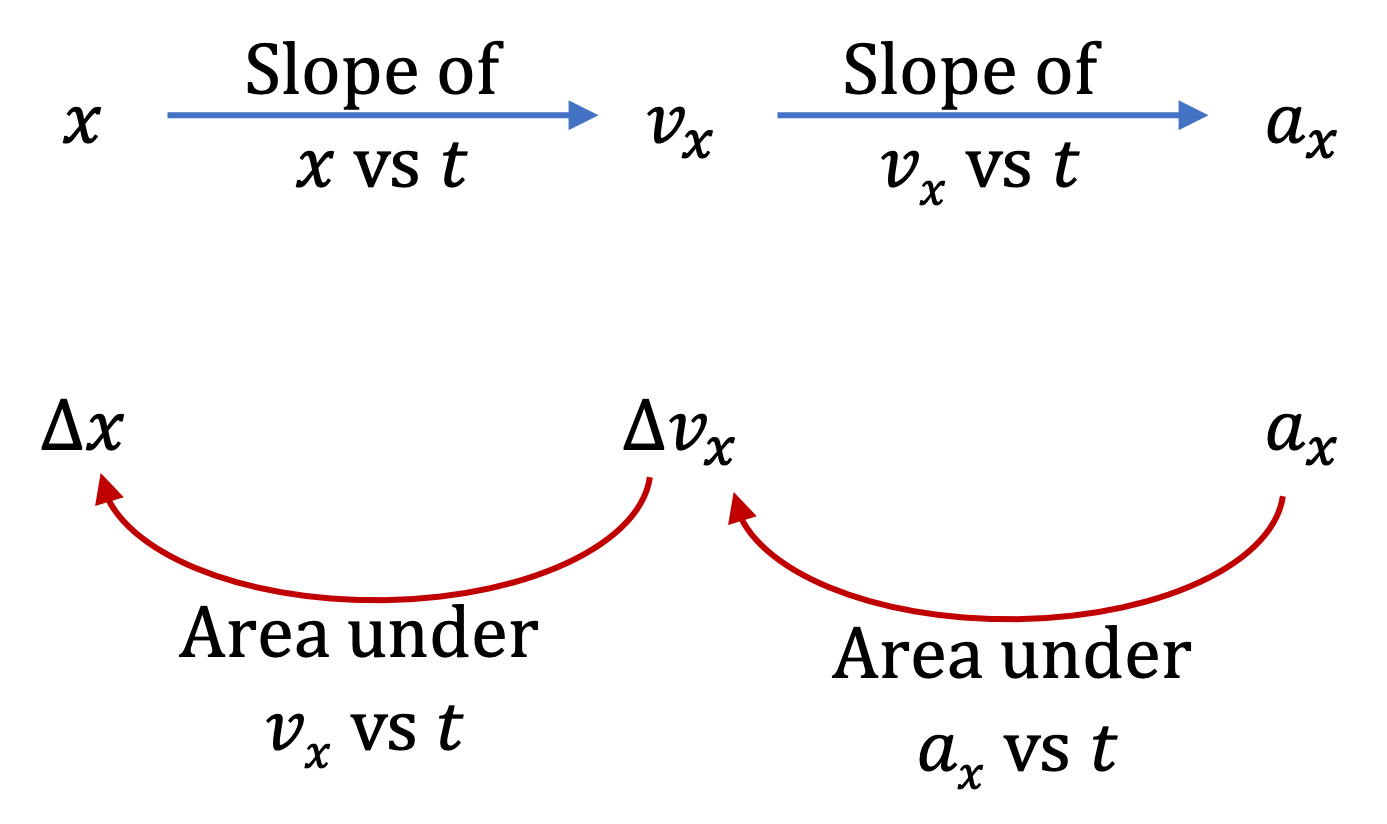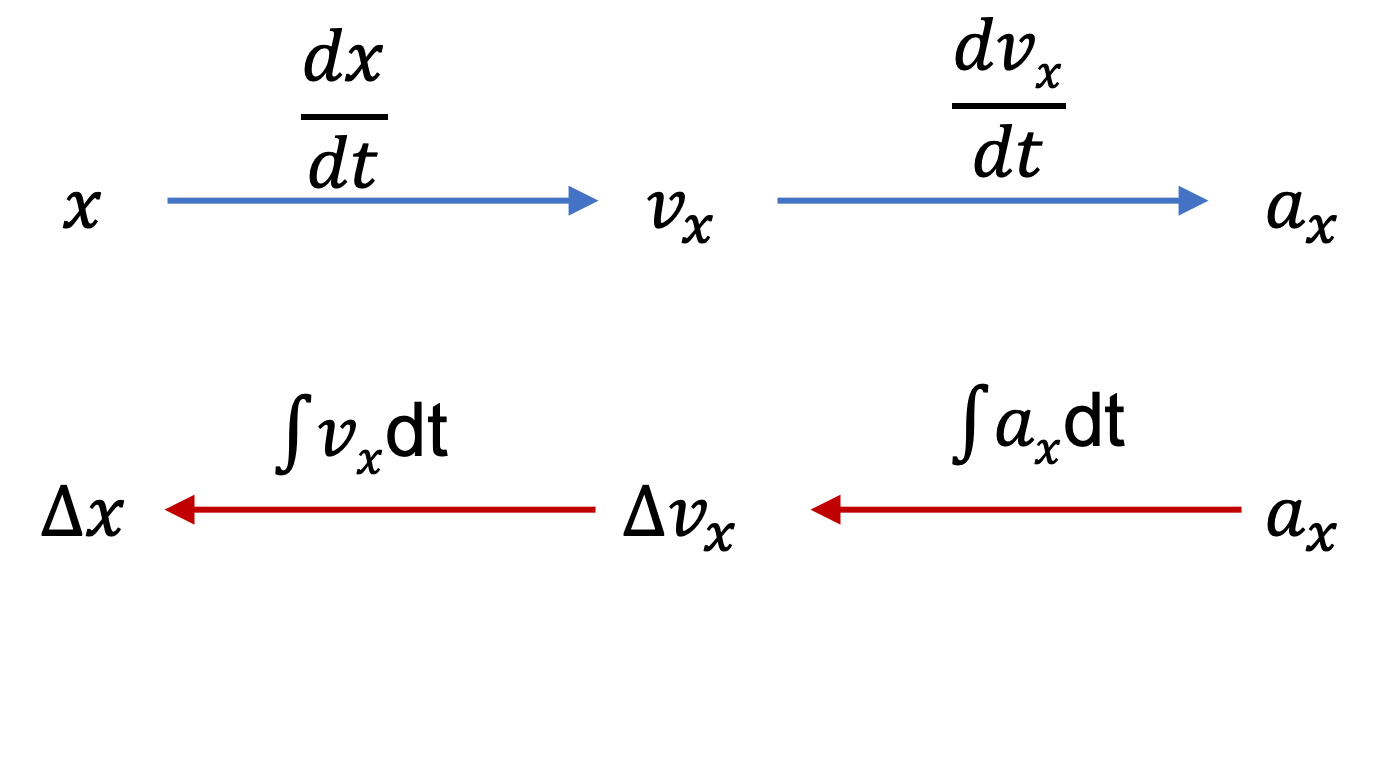We have covered a lot of ground and it is easy to get overwhelmed. In the end, we are basically studying a simple motion along $x$-axis and trying to quantify the rates of change of position $x$ and velocity $v_x\text{.}$ Figure 2.6.1 summarizes how to go from $x$ to $v_x$ to $a_x \text{,}$ and in the reverse order, from $a_x$ to $v_x$ to $x \text{.}$Figure 2.6.1. Relations in one-dimensional motion. We compute rate of change from the slopes of tangents of the quantity, and to compute the change in a quantity, we find area under the curve of the rate curve. For instance, to get the rate of change of $x\text{,}$ i.e., to compute $v_x\text{,}$ we find the slope of the tangent to the plot of $x$ versus $t \text{.}$ This is equivalent to taking the derivative of$x(t)\text{.}$ Similarly, the rate of change of $v_x\text{,}$ i.e., $a_x$ will come from the slope of the tangent to the plot of $v_x$ versus $t \text{,}$ which is equivalent to taking the derivative of$v_x(t)\text{.}$ Going in the reverse order, to compute the change in $x$ from $v_x$ versus $t$ plot, we compute the area under/over the curve, and similarly, to compute the change in $v_x$ from $a_x$ versus $t$ plot, we compute the area under/over the curve of $a_x\text{.}$ The area under the curve is another way of computing integrals.
The same schematic picture in Calculus language is shown in Figure 2.6.2.Figure 2.6.2. Schematics of relation between $x\text{,}$ corresponding velocity $v_x$ and acceleration $a_x\text{.}$